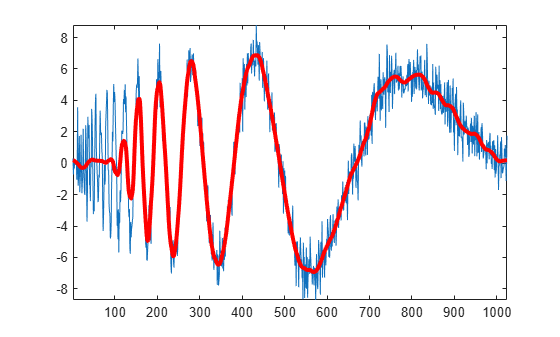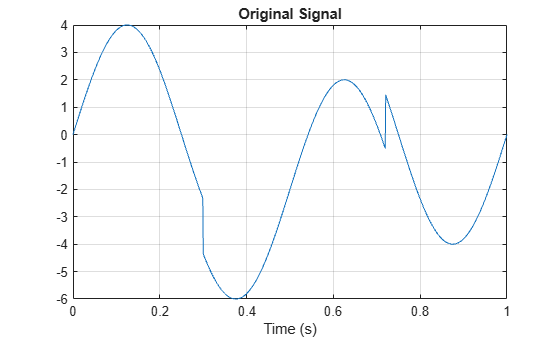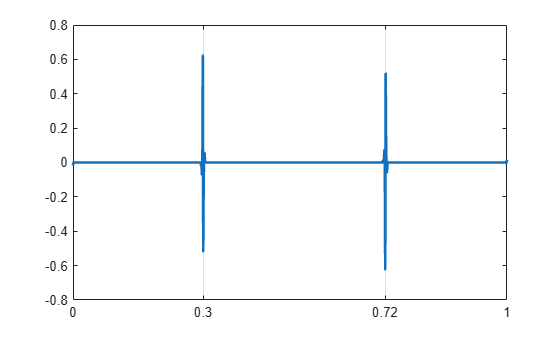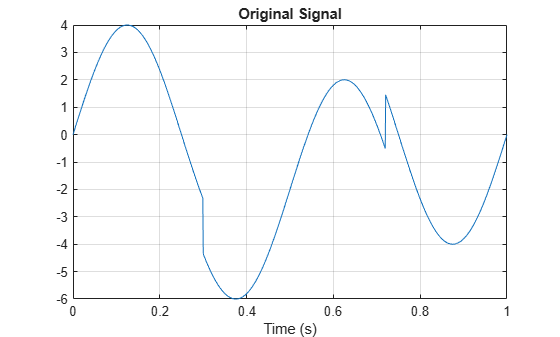dddtree

Dual-tree and double-density 1-D wavelet transform

Description

example

wt = dddtree(typetree,x,level,fdf,df) returns the typetree discrete wavelet transform (DWT) of the 1-D input signal, x, down to level, level. The wavelet transform uses the decomposition (analysis) filters, fdf, for the first level and the analysis filters, df, for subsequent levels. Supported wavelet transforms are the critically sampled DWT, double-density, dual-tree complex, and dual-tree double-density complex wavelet transform. The critically sampled DWT is a filter bank decomposition in an orthogonal or biorthogonal basis (nonredundant). The other wavelet transforms are oversampled filter banks.

example

wt = dddtree(typetree,x,level,fname) uses the filters specified by fname to obtain the wavelet transform. Valid filter specifications depend on the type of wavelet transform. See dtfilters for details.

example

wt = dddtree(typetree,x,level,fname1,fname2) uses the filters specified in fname1 for the first stage of the dual-tree wavelet transform and the filters specified in fname2 for subsequent stages of the dual-tree wavelet transform. Specifying different filters for stage 1 is valid and necessary only when typetree is 'cplxdt' or 'cplxdddt'.

Examples

collapse all

Obtain the complex dual-tree wavelet transform of the noisy Doppler signal. The FIR filters in the first and subsequent stages result in an approximately analytic wavelet as required.

Use dtfilters to create the first-stage Farras analysis filters and 6-tap Kingsbury Q-shift analysis filters for the subsequent stages of the multiresolution analysis.

df = dtfilters('dtf1');

The Farras and Kingsbury filters are in df{1} and df{2}, respectively. Load the noisy Doppler signal and obtain the complex dual-tree wavelet transform down to level 4.

wt = dddtree('cplxdt',noisdopp,4,df{1},df{2});

Plot an approximation based on the level-four approximation coefficients.

xapp = dddtreecfs('r',wt,'scale',{5});
plot(noisdopp)
hold on
plot(cell2mat(xapp),'r','linewidth',3)
axis tightUsing the output of dtfilters, or the filter name itself, in dddtree is preferable to manually entering truncated filter coefficients. To demonstrate the negative impact on signal reconstruction, create truncated versions of the Farras and Kingsbury analysis filters. Display the differences between the truncated and original filters.

Faf{1} = [0         0
-0.0884   -0.0112
0.0884    0.0112
0.6959    0.0884
0.6959    0.0884
0.0884   -0.6959
-0.0884    0.6959
0.0112   -0.0884
0.0112   -0.0884
0         0];
Faf{2} = [ 0.0112  0
0.0112         0
-0.0884   -0.0884
0.0884   -0.0884
0.6959    0.6959
0.6959   -0.6959
0.0884    0.0884
-0.0884    0.0884
0    0.0112
0   -0.0112];

af{1} = [ 0.0352         0
0         0
-0.0883   -0.1143
0.2339         0
0.7603    0.5875
0.5875   -0.7603
0    0.2339
-0.1143    0.0883
0         0
0   -0.0352];

af{2} = [0   -0.0352
0         0
-0.1143    0.0883
0    0.2339
0.5875   -0.7603
0.7603    0.5875
0.2339         0
-0.0883   -0.1143
0         0
0.0352         0];

max(max(abs(df{1}{1}-Faf{1})))
ans = 2.6792e-05
max(max(abs(df{1}{2}-Faf{2})))
ans = 2.6792e-05
max(max(abs(df{2}{1}-af{1})))
ans = 3.6160e-05
max(max(abs(df{2}{2}-af{2})))
ans = 3.6160e-05

Obtain the complex dual-tree wavelet transform down to level 4 using the truncated filters. Take the inverse transform and compare the reconstruction with the original signal.

wt = dddtree('cplxdt',noisdopp,4,Faf,af);
xrec = idddtree(wt);
max(abs(noisdopp-xrec))
ans = 0.0024

Do the same using the filter name. Confirm the difference is smaller.

wt = dddtree('cplxdt',noisdopp,4,'dtf1');
xrec = idddtree(wt);
max(abs(noisdopp-xrec))
ans = 2.1893e-07

Obtain the double-density wavelet transform of a signal with two discontinuities. Use the level-one detail coefficients to localize the discontinuities.

Create a signal consisting of a 2-Hz sine wave with a duration of 1 second. The sine wave has discontinuities at 0.3 and 0.72 seconds.

N = 1024;
t = linspace(0,1,1024);
x = 4*sin(4*pi*t);
x = x - sign(t - .3) - sign(.72 - t);
plot(t,x)
xlabel('Time (s)')
title('Original Signal')
grid onObtain the double-density wavelet transform of the signal. Reconstruct an approximation based on the level-one detail coefficients by first setting the lowpass (scaling) coefficients equal to 0. Plot the result. Observe features in the reconstruction align with the signal discontinuities.

wt = dddtree('ddt',x,1,'filters1');
wt.cfs{2} = zeros(1,512);
xrec = idddtree(wt);
plot(t,xrec,'linewidth',2)
set(gca,'xtick',[0 0.3 0.72 1])
set(gca,'xgrid','on')Obtain the complex dual-tree wavelet transform of a signal with two discontinuities. Use the first-level detail coefficients to localize the discontinuities.

Create a signal consisting of a 2-Hz sine wave with a duration of 1 second. The sine wave has discontinuities at 0.3 and 0.72 seconds.

N = 1024;
t = linspace(0,1,1024);
x = 4*sin(4*pi*t);
x = x - sign(t - .3) - sign(.72 - t);
plot(t,x)
xlabel('Time (s)')
title('Original Signal')
grid onObtain the dual-tree wavelet transform of the signal, reconstruct an approximation based on the level-one detail coefficients, and plot the result.

wt = dddtree('cplxdt',x,1,'FSfarras','qshift06');
wt.cfs{2} = zeros(1,512,2);
xrec = idddtree(wt);
plot(t,xrec,'linewidth',2)
set(gca,'xtick',[0 0.3 0.72 1])
set(gca,'xgrid','on')Input Arguments

collapse all

Type of wavelet decomposition, specified as one of 'dwt', 'ddt', 'cplxdt', or 'cplxdddt'. The type, 'dwt', gives a critically sampled (nonredundant) discrete wavelet transform. The other decomposition types produce oversampled wavelet transforms. 'ddt' produces a double-density wavelet transform. 'cplxdt' produces a dual-tree complex wavelet transform. 'cplxdddt' produces a double-density dual-tree complex wavelet transform.

Input signal, specified as an even-length row or column vector. If L is the value of the level of the wavelet decomposition, 2L must divide the length of x. Additionally, the length of the signal must be greater than or equal to the product of the maximum length of the decomposition (analysis) filters and 2(L-1).

Data Types: double

Level of the wavelet decomposition, specified as an integer. If L is the value of level, 2L must divide the length of x . Additionally, the length of the signal must be greater than or equal to the product of the maximum length of the decomposition (analysis) filters and 2(L-1).

Data Types: double

The level-one analysis filters, specified as a matrix or cell array of matrices. Specify fdf as a matrix when typetree is 'dwt' or 'ddt'. The size and structure of the matrix depend on the typetree input as follows:

• 'dwt' — This is the critically sampled discrete wavelet transform. In this case, fdf is a two-column matrix with the lowpass (scaling) filter in the first column and the highpass (wavelet) filter in the second column.

• 'ddt' — This is the double-density wavelet transform. The double-density DWT is a three-channel perfect reconstruction filter bank. fdf is a three-column matrix with the lowpass (scaling) filter in the first column and the two highpass (wavelet) filters in the second and third columns. In the double-density wavelet transform, the single lowpass and two highpass filters constitute a three-channel perfect reconstruction filter bank. This is equivalent to the three filters forming a tight frame. You cannot arbitrarily choose the two wavelet filters in the double-density DWT. The three filters together must form a tight frame.

Specify fdf as a 1-by-2 cell array of matrices when typetree is a dual-tree transform, 'cplxdt' or 'cplxdddt'. The size and structure of the matrix elements depend on the typetree input as follows:

• For the dual-tree complex wavelet transform, 'cplxdt', fdf{1} is a two-column matrix containing the lowpass (scaling) filter and highpass (wavelet) filters for the first tree. The scaling filter is the first column and the wavelet filter is the second column. fdf{2} is a two-column matrix containing the lowpass (scaling) and highpass (wavelet) filters for the second tree. The scaling filter is the first column and the wavelet filter is the second column.

• For the double-density dual-tree complex wavelet transform, 'cplxdddt', fdf{1} is a three-column matrix containing the lowpass (scaling) and two highpass (wavelet) filters for the first tree and fdf{2} is a three-column matrix containing the lowpass (scaling) and two highpass (wavelet) filters for the second tree.

Data Types: double

Analysis filters for levels > 1, specified as a matrix or cell array of matrices. Specify df as a matrix when typetree is 'dwt' or 'ddt'. The size and structure of the matrix depend on the typetree input as follows:

• 'dwt' — This is the critically sampled discrete wavelet transform. In this case, df is a two-column matrix with the lowpass (scaling) filter in the first column and the highpass (wavelet) filter in the second column. For the critically sampled orthogonal or biorthogonal DWT, the filters in df and fdf must be identical.

• 'ddt' — This is the double-density wavelet transform. The double-density DWT is a three-channel perfect reconstruction filter bank. df is a three-column matrix with the lowpass (scaling) filter in the first column and the two highpass (wavelet) filters in the second and third columns. In the double-density wavelet transform, the single lowpass and two highpass filters must constitute a three-channel perfect reconstruction filter bank. This is equivalent to the three filters forming a tight frame. For the double-density DWT, the filters in df and fdf must be identical.

Specify df as a 1-by-2 cell array of matrices when typetree is a dual-tree transform, 'cplxdt' or 'cplxdddt'. For dual-tree transforms, the filters in fdf and df must be different. The size and structure of the matrix elements in the cell array depend on the typetree input as follows:

• For the dual-tree complex wavelet transform, 'cplxdt', df{1} is a two-column matrix containing the lowpass (scaling) and highpass (wavelet) filters for the first tree. The scaling filter is the first column and the wavelet filter is the second column. df{2} is a two-column matrix containing the lowpass (scaling) and highpass (wavelet) filters for the second tree. The scaling filter is the first column and the wavelet filter is the second column.

• For the double-density dual-tree complex wavelet transform, 'cplxdddt', df{1} is a three-column matrix containing the lowpass (scaling) and two highpass (wavelet) filters for the first tree and df{2} is a three-column matrix containing the lowpass (scaling) and two highpass (wavelet) filters for the second tree.

Data Types: double

Filter name, specified as a character vector or string scalar. For the critically sampled DWT, specify any valid orthogonal or biorthogonal wavelet filter. See wfilters for details. For the double-density wavelet transform, 'ddt', valid choices are 'filters1' and 'filters2'. For the complex dual-tree wavelet transform, valid choices are 'dtfP' with P = 1, 2, 3, 4. For the double-density dual-tree wavelet transform, the only valid choice is 'dddtf1'. See dtfilters for more details on valid filter names for the oversampled wavelet filter banks.

Data Types: char

First-stage filter name, specified as a character vector or string scalar. Specifying a different filter for the first stage is valid and necessary only in the dual-tree transforms, 'cplxdt' and 'cplxddt'. In the complex dual-tree wavelet transform, you can use any valid wavelet filter for the first stage. In the double-density dual-tree wavelet transform, the first-stage filters must form a three-channel perfect reconstruction filter bank.

Data Types: char

Filter name for stages > 1, specified as a character vector or string scalar. You must specify a first-level filter that is different from the wavelet and scaling filters in subsequent levels when using the dual-tree wavelet transforms, 'cplxdt' or 'cplxdddt'. See dtfilters for valid choices.

Data Types: char

Output Arguments

collapse all

Wavelet transform, returned as a structure with these fields:

Type of wavelet decomposition (filter bank) used in the analysis, returned as one of 'dwt', 'ddt', 'cplxdt', or 'cplxdddt'. The type, 'dwt', gives a critically sampled discrete wavelet transform. The other types correspond to oversampled wavelet transforms. 'ddt' is a double-density wavelet transform, 'cplxdt' is a dual-tree complex wavelet transform, and 'cplxdddt' is a double-density dual-tree complex wavelet transform.

Level of wavelet decomposition, returned as a positive integer.

Decomposition (analysis) and reconstruction (synthesis) filters, returned as a structure with these fields:

First-stage analysis filters, returned as an N-by-2 or N-by-3 matrix for single-tree wavelet transforms, or a cell array of two N-by-2 or N-by-3 matrices for dual-tree wavelet transforms. The matrices are N-by-3 for the double-density wavelet transforms. For an N-by-2 matrix, the first column of the matrix is the scaling (lowpass) filter and the second column is the wavelet (highpass) filter. For an N-by-3 matrix, the first column of the matrix is the scaling (lowpass) filter and the second and third columns are the wavelet (highpass) filters. For the dual-tree transforms, each element of the cell array contains the first-stage analysis filters for the corresponding tree.

Analysis filters for levels > 1, returned as an N-by-2 or N-by-3 matrix for single-tree wavelet transforms, or a cell array of two N-by-2 or N-by-3 matrices for dual-tree wavelet transforms. The matrices are N-by-3 for the double-density wavelet transforms. For an N-by-2 matrix, the first column of the matrix is the scaling (lowpass) filter and the second column is the wavelet (highpass) filter. For an N-by-3 matrix, the first column of the matrix is the scaling (lowpass) filter and the second and third columns are the wavelet (highpass) filters. For the dual-tree transforms, each element of the cell array contains the analysis filters for the corresponding tree.

First-level reconstruction filters, returned as an N-by-2 or N-by-3 matrix for single-tree wavelet transforms, or a cell array of two N-by-2 or N-by-3 matrices for dual-tree wavelet transforms. The matrices are N-by-3 for the double-density wavelet transforms. For an N-by-2 matrix, the first column of the matrix is the scaling (lowpass) filter and the second column is the wavelet (highpass) filter. For an N-by-3 matrix, the first column of the matrix is the scaling (lowpass) filter and the second and third columns are the wavelet (highpass) filters. For the dual-tree transforms, each element of the cell array contains the first-stage synthesis filters for the corresponding tree.

Reconstruction filters for levels > 1, returned as an N-by-2 or N-by-3 matrix for single-tree wavelet transforms, or a cell array of two N-by-2 or N-by-3 matrices for dual-tree wavelet transforms. The matrices are N-by-3 for the double-density wavelet transforms. For an N-by-2 matrix, the first column of the matrix is the scaling (lowpass) filter and the second column is the wavelet (highpass) filter. For an N-by-3 matrix, the first column of the matrix is the scaling (lowpass) filter and the second and third columns are the wavelet (highpass) filters. For the dual-tree transforms, each element of the cell array contains the synthesis filters for the corresponding tree.

Wavelet transform coefficients, returned as a 1-by-(level+1) cell array of matrices. The size and structure of the matrix elements of the cell array depend on the type of wavelet transform, typetree, as follows:

• 'dwt'cfs{j}

• j = 1,2,... level is the level.

• cfs{level+1} are the lowpass, or scaling, coefficients.

• 'ddt'cfs{j}(:,:,k)

• j = 1,2,... level is the level.

• k = 1,2 is the wavelet filter.

• cfs{level+1}(:,:) are the lowpass, or scaling, coefficients.

• 'cplxdt'cfs{j}(:,:,m)

• j = 1,2,... level is the level.

• m = 1,2 are the real and imaginary parts.

• cfs{level+1}(:,:,m) are the lowpass, or scaling, coefficients.

• 'cplxdddt'cfs{j}(:,:,k,m)

• j = 1,2,... level is the level.

• k = 1,2 is the wavelet filter.

• m = 1,2 are the real and imaginary parts.

• cfs{level+1}(:,:,m) are the lowpass, or scaling, coefficients.

Introduced in R2013b

Wavelet Toolbox DocumentationGet trial now